# Solving a TSP with Linear Programming and Google OR-Tools in PythonIn this post I show you how to solve the TSP problem using integer linear programming and Google OR-Tools for mathmatical modelling in Python. If you’re not yet familiar to the TSP and want to dig deeper, find out more here. It is one of the oldest and best explored problems in the field of Operations Research. In previous posts I have already presented two ways of solving the TSP using heuristic approaches: Solve TSP using Pilot Method and Solve TSP Instance using Google OR-Tools. In this post we will see a solution approach based on mathematical programming to always find the optimal solution.

## The Problem

The TSP goes as follows: We have a set of locations, i.e. given by geo-coordinates, which we all want to visit in a single trip. Each location must exactly be visited once, the tour must end at the same location where it started and the sense doesn’t matter. The objective is to find the shortest trip.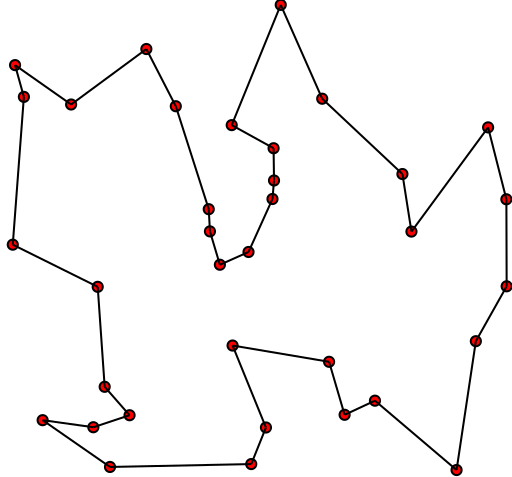Example for a TSP trip (by Xypron, public domain)

In real life we typically woud have a set of adresses for all locations. However, what we need is a distance matrix providing the distance in km for any pair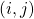of locations. We could use an OSRM server set up with open street map material of the desired country in order to first geocode the adresses into pairs of lat/long coordinates and then finally generate a distance matrix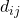.

However, since generating distance matrices is not the main focus here, we’ll take a TSP problem provided in the standardized TSP fileformat and use a simple command to convert it to a spatial distance-matrix.

## The Model

Let’s start to model the problem as an Mixed Integer Linear Program (MIP). When designing an MIP, we usually follow the following recipe:

1. Choose the decision variable
2. Choose the auxiliary variables (if required)
3. Define the indices and sets (if required)
4. Define the parameters
5. Define the constraints
6. Define the objective function
7. Validate the model

### 1) Choose the Decision Variable

In order to define a sequence of visits among the points, we typically use a decisionvariable carrying two indices i and j. This allows to model relations between any two locations, which turns out practical. Remember: We need to be able to refer to the previous or the following point of a TSP tour, to model relations.

Therefore we define the decision variable as follows: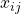= 1, if location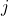is visited in our TSP trip just after location, 0 otherwise.

### 2) Choose the Auxiliary Variables

At the moment, we don’t yet have a need for auxiliary variables.

### 3) Define the Indices and Sets

We have two indices,and, both are coming from the same set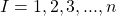with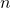being the number of locations to be visited in our TSP.

### 4) Define the Parameters

The only parameter we need here is the distance matrixfrom above, providing the distance in km between locationand. Since our problem is symmetrical, the vaues in the distance matrix are symmetrical with respect to the diagonal (lower / upper triangular matrix are identical).

### 5) Define the Constraints

• Leave every point for exactly one successor: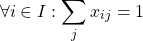• Reach every point from exactly one predecessor:At first sight, these constraints might seem to be complete. For now, let’s leave it that way. We’ll comeback to this later, when following the 7-step recipe presented above.

### 6) Objective Function

Since we want to minimize the total distance, the objective function is straight forward: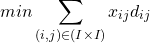We just have to minimize the sum of all sections in the trip.

### 7) Validate the Model

Now let’s validate the model. This is often done first on a purely logical level. For example, we can check whether the model handles certain special cases or boundary conditions correctly.

The two conditions mean that each location must be left and approached once. This leads to closed tours, since it’s not allowed to end or start in a location, without returning to it. However, it is not forbidden to multiple closed tours. We have already discussed this fact in more detail in this post. Here we realize that our model is still incomplete, as it allows impermissible constellations.

So our model failed the logical test. We are expanding it to avoid this inadequacy. Let’s revise our model and introduce the missing subtour elimination constraint (details you can read here):

### 2) Choose the auxiliary Variables

The Miller-Tucker-Zemlin subtour elimination constraint requires an additional decision variable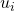to enumerate the stops in sequential order.

### 5) Define the Constraints

Now based on the new auxiliary variablewe can formulate the subtour elimination constraints. Since it doesn’t matter, where to start, let’s start at the first location: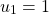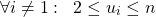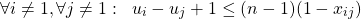Since we have n locations, all subsequentmust have assigned a vaule between 2 and. The last constraint makes sure, that the index of every succeeding stop gets assigned an index which is at least 1 larger than the predecessor’s index. Together with the above condition, each successor can only be assigned an index that is exactly one greater than that of its predecessor.

### 7) Validate the Model

Now let’s validate the model once more. From the logical standpoint of view, the model seems to be correct and complete now. Now it’s time to check wheter the model solves the problem instances as expected in practice.

Hint: The TSP is one of the hardest combinatorial problems and the model presented here allows to find optimal solutions. However, it’s by far not the best model, since the integer relaxation of the MTZ-constraint is very weak. Even one of the smallest national problem instances provided in the university of waterloo, the Djibouti – instance, makes gurobi – the world’s best MIP solver – sweat!

## The Code

Now let’s implement the solution in python. The cool thing is, that using google or-tools we have a powerful tool at hand to translate the model into code. Let’s first see, how to do this:

    # constraint 1: leave every point exactly once
log.info('Creating ' + str(num_nodes) + ' Constraint 1... ')
for i in all_nodes:
model.Add(sum(x[(i, j)] for j in all_nodes) == 1)

As we can see, that the constraint can easily be translated. The for-all clause ends up in the loop, the sigma-formula is translated directly with binding the other left indices using for … in … clauses and the term can be take exactly from the formal constraint definition.

Let’s translate the other constraints:

    # constraint 2: reach every point from exactly one other point
log.info('Creating ' + str(num_nodes) + ' Constraint 2... ')
for j in all_nodes:
model.Add(sum(x[(i, j)] for i in all_nodes) == 1)

# constraint 3.1: subtour elimination constraints (Miller-Tucker-Zemlin) part 1
log.info('Creating 1 Constraint 3.1... ')

# constraint 3.2: subtour elimination constraints (Miller-Tucker-Zemlin) part 2
log.info('Creating ' + str(len(all_but_first_nodes)) + ' Constraint 3.2... ')
for i in all_but_first_nodes:

# constraint 3.3: subtour elimination constraints (Miller-Tucker-Zemlin) part 3
log.info('Creating ' + str(len(all_but_first_nodes)) + ' Constraint 3.2... ')
for i in all_but_first_nodes:
for j in all_but_first_nodes:
model.Add(u[i] - u[j] + 1 <= (num_nodes - 1) * (1 - x[(i, j)]))

Finally we have to translate the objective function:

    # Minimize the total distanceg der Anzahl geänderte Dienste und Anzahl Dienstfahrten
model.Minimize(sum(x[(i, j)] * dima[(i, j)] for i in all_nodes for j in all_nodes))

That’s it! The rest of the code is about loading the TSP data, generating the distance matrix, calling the solver – i.e. gurobi – to solve the model and then output the solution:

from builtins import min

import scipy.spatial as sp
import pandas as pd
import numpy as np
import logging as log
import json
import sys
# also required packages: openpyxl
from ortools.linear_solver import pywraplp

def solve_OrTools(dima):
'''
generate mip model using google or-tools and solve it

:param dima: the distance matrix
:return:  solution X, model, status
'''

if dima.ndim != 2 or dima.shape != dima.shape:
raise ValueError("Invalid dima dimensions detected. Square matrix expected.")

# determine number of nodes
num_nodes = dima.shape
all_nodes = range(0, num_nodes)
all_but_first_nodes = range(1, num_nodes)

# Create the model.
solver_name = 'GUROBI_MIP'
log.info('Instantiating solver ' + solver_name)
model = pywraplp.Solver.CreateSolver(solver_name)
model.EnableOutput()

log.info('Defining MIP model... ')
# generating decision variables X_ij
log.info('Creating ' + str(num_nodes * num_nodes) + ' boolean x_ij variables... ')
x = {}
for i in all_nodes:
for j in all_nodes:
x[(i, j)] = model.BoolVar('x_i%ij%i' % (i, j))

log.info('Creating ' + str(num_nodes) + ' boolean u_i variables... ')
u = {}
for i in all_nodes:
u[i] = model.IntVar(0, num_nodes, 'u_i%i' % i)

# constraint 1: leave every point exactly once
log.info('Creating ' + str(num_nodes) + ' Constraint 1... ')
for i in all_nodes:
model.Add(sum(x[(i, j)] for j in all_nodes) == 1)

# constraint 2: reach every point from exactly one other point
log.info('Creating ' + str(num_nodes) + ' Constraint 2... ')
for j in all_nodes:
model.Add(sum(x[(i, j)] for i in all_nodes) == 1)

# constraint 3.1: subtour elimination constraints (Miller-Tucker-Zemlin) part 1
log.info('Creating 1 Constraint 3.1... ')

# constraint 3.2: subtour elimination constraints (Miller-Tucker-Zemlin) part 2
log.info('Creating ' + str(len(all_but_first_nodes)) + ' Constraint 3.2... ')
for i in all_but_first_nodes:

# constraint 3.3: subtour elimination constraints (Miller-Tucker-Zemlin) part 3
log.info('Creating ' + str(len(all_but_first_nodes)) + ' Constraint 3.2... ')
for i in all_but_first_nodes:
for j in all_but_first_nodes:
model.Add(u[i] - u[j] + 1 <= (num_nodes - 1) * (1 - x[(i, j)]))

# Minimize the total distance
model.Minimize(sum(x[(i, j)] * dima[(i, j)] for i in all_nodes for j in all_nodes))

log.info('Solving MIP model... ')
status = model.Solve()

return u, model, status

def print_solution(u):
num_nodes = len(u)
all_nodes = range(0, num_nodes)
for i in all_nodes:
log.info('u(' + str(i) + ')=' + str(int(u[i].solution_value())))

def main():
# configure logger for info level
log.basicConfig(
format='%(asctime)s %(levelname)-8s %(message)s',
level=log.INFO,
datefmt='%Y-%m-%d %H:%M:%S',
stream=sys.stdout)

# load tsp instance
tsp_problem = 'dj10.tsp'

log.info("Reading TSP problem Instance " + tsp_problem)
tsp = pd.read_csv('./TSP_Instances/' + tsp_problem, sep=' ', skiprows=10, dtype = float,
names=['nodeId', 'lat', 'lng'], skipfooter=0, engine='python')
tsp = tsp.sort_values(by='nodeId', inplace=False)

A = tsp[['lat', 'lng']].to_numpy()
dima = sp.distance_matrix(A, A)

# now solve problem
u, model, status = solve_OrTools(dima)

# check problem response
if status == pywraplp.Solver.OPTIMAL:
log.info('Solution:')
log.info('optimal solution found.')
log.info('Objective value =' + str(model.Objective().Value()))
print_solution(u)
elif status == pywraplp.Solver.INFEASIBLE:
log.info('The problem is infeasible.')
else:
log.info('The problem could not be solved. Return state was: ' + status)

# Press the green button in the gutter to run the script.
if __name__ == '__main__':
main()


The complete source together with the Djabouti instance can be downloaded from my github account here. I used gurobi here as a solver. There are plenty of other solvers you can use here, some of which are provided by google’s or-tools, others are provided from other open source projects and published under vairous licenses.

If you want to use another solver, just install it on your pc ans replace the solver name provided in the source above. If you don’t have gurobi, try solver_name = ‘SCIP’. This is an open source solver, published under the GNU license.

Categories: